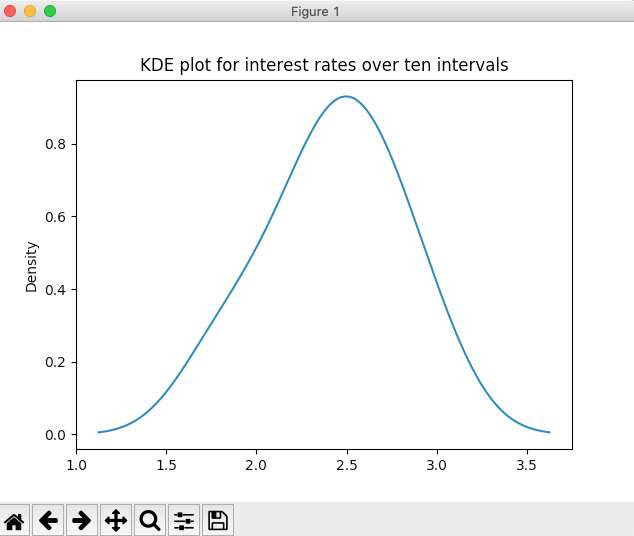# Drawing Kernel Density Estimation-KDE Plot Using Pandas Series

## Overview:

• The kernel density estimation plot draws the probability density for a given distribution.
• The kernel density plot provides vital display of information on data which include:
• How the data is distributed around the measures of central tendency like mean and median
• How the distribution is skewed
• How the distribution is peaked
• For a distribution present in a pandas Series, the kernel density estimation plot is drawn by calling the function kde() on the plot member of the Series instance.
• In the same way to plot the kernel density estimation plot for a pandas DataFrame the function kde() can be invoked on the DataFrame.plot member.

## Example:

 # Example Python program to draw a # Kernel Density Estimation plot # for a pandas Series   import pandas as pds import matplotlib.pyplot as plt   # Interest rate data as a Python list distribution    = [2.0, 1.75, 2.25, 2.5, 2.25, 2.5, 2.75, 3, 2.75, 2.5]; series          = pds.Series(distribution);   # Draw a KDE plot series.plot.kde(title="KDE plot for interest rates over ten intervals");   plt.show(block=True);

## Output: